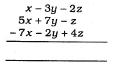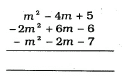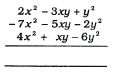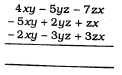# RS Aggarwal Solutions Class 6 Ex 8A

## RS Aggarwal Class 6 Ex 8A Chapter 8

1) Add:

(i) 3x, 7x

Required sum = 3x + 7x

= (3+7)x

= 10x

(ii) 7y, -9y

Required sum = 7y +(-9y)

= (7-9)y

= -2y

(iii) 2xy, 5xy, -xy

Required sum = 2xy +5xy + (-xy)

= (2+5-1)xy

= 6xy

(iv) 3x, 2y

Required sum = 3x+2y

(v) 2$x^{2}$,- 3$x^{2}$,7$x^{2}$

Required sum = 2$x^{2}$ + (- 3$x^{2}$) + 7$x^{2}$

=(2-3+7) $x^{2}$

= 6$x^{2}$

(vi) 7xyz ,- 5xyz, 9xyz, -8xyz

Required sum = 7xyz + (- 5xyz) + 9xyz + (-8xyz)

= (7-5+9-8) xyz

= 3xyz

(vii) 6$a^{3}$, – 4$a^{3}$, 10$a^{3}$ , -8$a^{3}$

Required sum = 6$a^{3}$ +(- 4$a^{3}$) + 10$a^{3}$ +( -8$a^{3}$)

=(6-4+10-8) $a^{3}$

= 4$a^{3}$

(viii) $x^{2}$$a^{2}$, -5$x^{2}$ + 2$a^{2}$), – 4$x^{2}$ + 4$a^{2}$

Required sum = $x^{2}$$a^{2}$ + (-5$x^{2}$ + 2$a^{2}$) +( – 4$x^{2}$ + 4$a^{2}$ )

Rearranging and collecting the like terms = $x^{2}$ -5$x^{2}$ – 4$x^{2}$$a^{2}$ + 2$a^{2}$ + 4$a^{2}$

= (1-5-4) $x^{2}$ + (-1+2+4) $a^{2}$

= -8$x^{2}$ + 5$a^{2}$

2)

(i)x- 3y -2z

5 x + 7y – z

-7x – 2y + 4z

-x +2y + z

(ii)$m^{2}$ – 4m + 5 – 2$m^{2}$ + 6m – 6 – $m^{2}$ – 2m – 7 -2$m^{2}$ +0x m-8 = -2$m^{2}$ – 8 = -2$m^{2}$ – 8

(iii)2 x 2 – 3xy + $y^{2}$ – 7$x^{2}$ – 5xy – 2$y^{2}$ 4$x^{2}$ + xy – 6$y^{2}$$x^{2}$ -7xy -7$y^{2}$

(iv)4xy – 5yz – 7zx- 5xy + 2yz + zx- 2xy – 3yz + 3zx-3xy -6yz -3zx

3) Add:

(i) (3a – 2b + 5c), (2a + 5b – 7c), (- a – b + c)

Sum of the given expressions

= (3a – 2b + 5c) + (2a + 5b – 7c) + (- a – b + c)

Rearranging and collecting the like terms

= 3a + 2a – a – 2b + 5b – b + 5c – 7c + c

= (3+2-1)a + (-2+5-1)b + (5-7+1)c

= 4a+2b-c

(ii) Sum of the given expressions

(8a – 6ab + 5b), (- 6a – ab – 8b), (-4a + 2ab + 3b)

= (8a – 6ab + 5b) + (- 6a – ab – 8b) + (-4a + 2ab + 3b)

Rearranging and collecting the like terms

= (8 – 6 – 4 )a + (- 6 – 1 + 2)ab + (5 – 8 + 3)b

= -2a – 5ab + 0

= -2a – 5ab

(iii) 2$x^{3}$ – 3$x^{2}$ + 7x – 8,-5$x^{3}$ + 2$x^{2}$ – 4x + 1, 3 – 6x + 5$x^{2}$$x^{3}$

Sum of the given expressions

= (2$x^{3}$ – 3$x^{2}$ + 7x – 8) + (-5$x^{3}$ + 2$x^{2}$ – 4x + 1) + ( 3 – 6x + 5×2 – x3) Rearranging and collecting the like terms =2×3-5×3 – x3 – 3×2 + 2×2 + 5×2 +7x-4x-6x-8+1+3 = (2-5-1)x3 +(-3+2+5)x2+(7-4-G)x-4 = -4×3 +4×2-3x-4

(iv) 2$x^{2}$ – 8xy + 7$y^{2}$ – 8x$y^{2}$, 2x$y^{2}$ + 6xy – $y^{2}$ + 3$x^{2}$, 4$y^{2}$ – xy – $x^{2}$ + x$y^{2}$

Sum of the given expressions = (2$x^{2}$ – 8xy + 7$y^{2}$ – 8x$y^{2}$)+( 2x$y^{2}$ + 6xy – $y^{2}$ + 3$x^{2}$) + ( 4$y^{2}$ – xy – $x^{2}$ + x$y^{2}$ )

Rearranging and collecting the like terms

= 2$x^{2}$ +3$x^{2}$$x^{2}$ + 7$y^{2}$$y^{2}$ +4$y^{2}$ – 8xy + 6xy – xy – 8x$y^{2}$ +2x$y^{2}$ + x$y^{2}$

= (2 +3- 1) $x^{2}$ + (7 – 1 +4) $y^{2}$ + (-8 + 6 -1)xy + (- 8 +2 +1)x$y^{2}$

= 4$x^{2}$ + 10$y^{2}$ – 3xy -5x$y^{2}$

(v) $x^{3}$ + $y^{3}$$z^{3}$ + 3xyz, – $x^{3}$ + $y^{3}$ + $z^{3}$ – 6xyz, $x^{3}$$y^{3}$$z^{3}$ – 8xyz

Sum of the given expressions = ($x^{3}$ + $y^{3}$$z^{3}$ + 3xyz)+(- $x^{3}$ + $y^{3}$ + $z^{3}$ – 6xyz)+( $x^{3}$$y^{3}$$z^{3}$ – 8xyz)

Rearranging and collecting the like terms

= 6$x^{3}$$x^{3}$ + $x^{3}$ + $y^{3}$ + $y^{3}$$y^{3}$$z^{3}$ + $z^{3}$$z^{3}$ + 3xyz -6xyz – 8xyz

= (1-1+1) $x^{3}$ + (1+1-1) $y^{3}$ + (-1+1-1) $z^{3}$ +(3-6-8)xyz

= $x^{3}$ + ya – $z^{3}$ -11xyz

(vi) 2 + x – $x^{2}$ + 6$x^{3}$, -6 -2x + 4$x^{2}$ -3$x^{3}$, 2 + $x^{2}$, 3 – $x^{3}$ + 4x – 2$x^{2}$

Sum of the given expressions

= (2 + x – $x^{2}$ + 6$x^{3}$) + (-6 -2x + 4$x^{2}$ -3$x^{3}$)+( 2 + $x^{2}$)+( 3 – $x^{3}$ + 4x – 2$x^{2}$ )

Rearranging and collecting the like terms

= 6$x^{3}$ – 3$x^{3}$$x^{3}$$x^{2}$ + 4$x^{2}$ + $x^{2}$ – 2$x^{2}$ + x – 2x+ 4x+2 – 6+2+3

= (6-3-1) $x^{3}$ + (-1+4+1-2) $x^{2}$ +(1-2+4)x + 1

= 2$x^{3}$ +2$x^{2}$ +3x+1

4) Subtract:

Change the sign of each term of the expression that is to be subtracted and then add.

(i) 5x from 2x

Term to be subtracted = 5x

Changing the sign of each term of the expression gives -5x.

On adding:

2x + (-5x) = 2x-5x

= (2-5)x

= -3x

(ii) –xy from 6xy

Term to be subtracted = -xy

Changing the sign of each term of the expression gives xy.

On adding:

6xy + xy

= (6+1)xy

= 7xy

(iii) 3a from 5b

Term to be subtracted = 3a

Changing the sign of each term of the expression gives -3a.

On adding:

5b+(-3a) = 5b-3a

(iv) -7x from 9y

Term to be subtracted = -7x

Changing the sign of each term of the expression gives 7x.

On adding: 9y+7x

(v) 10$x^{2}$ from -7$x^{2}$

Term to be subtracted = 10$x^{2}$

Changing the sign of each term of the expression gives -10$x^{2}$.

On adding:

-7$x^{2}$ + (-10$x^{2}$) = -7$x^{2}$ -10$x^{2}$ = (-7-10) $x^{2}$ = -17$x^{2}$

(vi) $a^{2}$$b^{2}$ from $b^{2}$$a^{2}$

Term to be subtracted = $a^{2}$$b^{2}$

Changing the sign of each term of the expression gives –$a^{2}$$b^{2}$.

On adding:

$b^{2}$$a^{2}$ + (-$a^{2}$ + $b^{2}$) = $b^{2}$$a^{2}$$a^{2}$ + $b^{2}$

= (1+1) $b^{2}$ +(-1-1) $a^{2}$

= 2$b^{2}$ – 2$a^{2}$

5) Subtract:

Change the sign of each term of the expression that is to be subtracted and then add.

(i) 5a + 7b – 2c from 3a – 7b + 4c

Term to be subtracted = 5a + 7b – 2c

Changing the sign of each term of the expression gives -5a -7b + 2c.

On adding:

(3a – 7b + 4c) + (-5a -7b + 2c ) = 3a – 7b + 4c-5a -7b + 2c

= (3-5)a+( – 7-7)b + (4+2)c

= -2a – 14b + 6c

(ii) a – 2b – 3c from -2a + 5b – 4c

Term to be subtracted = a – 2b – 3c

Changing the sign of each term of the expression gives -a +2b + 3c.

On adding:

(-2a + 5b – 4c)+(-a +2b + 3c ) = -2a + 5b – 4c-a +2b + 3c

= (-2-1)a + (5+2)b +(-4+3)c

= -3a + 7b – c

(iii) 5$x^{2}$ – 3xy + $y^{2}$ from 7$x^{2}$ – 2xy – 4$y^{2}$

Term to be subtracted = 5$x^{2}$ – 3xy + $y^{2}$

Changing the sign of each term of the expression gives -5$x^{2}$ + 3xy – $y^{2}$.

On adding:

(7$x^{2}$ – 2xy – 4$y^{2}$)+(-5$x^{2}$ + 3xy – $y^{2}$)

= 7$x^{2}$ – 2xy – 4$y^{2}$ -5$x^{2}$ + 3xy – $y^{2}$

= (7-5) $x^{2}$ + (-2+3)xy +(-4-1) $y^{2}$

= 2$x^{2}$ + xy – 5$y^{2}$

(iv) 6$x^{3}$ – 7$x^{2}$ + 5x – 3 from 4 – 5x + 6$x^{2}$ – 8$x^{3}$

Term to be subtracted = 6$x^{3}$ – 7$x^{2}$ + 5x – 3

Changing the sign of each term of the expression gives -6$x^{3}$ + 7$x^{2}$ – 5x + 3.

On adding:

(4 – 5x + 6$x^{2}$ – 8$x^{3}$)+(-6$x^{3}$ + 7$x^{2}$ – 5x + 3)

= 4 – 5x + 6$x^{2}$ – 8$x^{3}$ -6$x^{3}$ + 7$x^{2}$ – 5x + 3

= (-8-6) $x^{3}$ +(6+7) $x^{2}$ +(-5- 5)x + 7

= -14$x^{3}$ + 13$x^{2}$ – 10x + 7

(v) $x^{3}$ + 2$x^{2}$ y + 6x$y^{2}$$y^{3}$ from ($y^{3}$ – 3x$y^{2}$ – 4$x^{2}$y

Term to be subtracted = $x^{3}$ + 2$x^{2}$ y + 6x$y^{2}$$y^{3}$

Changing the sign of each term of the expression gives

$x^{3}$ – 2$x^{2}$y – 6x$y^{2}$ + $y^{3}$.

On adding: ($y^{3}$ – 3x$y^{2}$ – 4$x^{2}$y) + (-$x^{3}$ – 2$x^{2}$ y – 6x$y^{2}$ + $y^{3}$)

= $y^{3}$ – 3x$y^{2}$ – 4$x^{2}$ y – $x^{3}$ – 2$x^{2}$ y – 6x$y^{2}$ + $y^{3}$

= – $x^{3}$ +(- 2-4) $x^{2}$ y + (-6-3)x$y^{2}$ + (1+1) $y^{3}$

= –$x^{3}$ – 6$x^{2}$ y – 9x$y^{2}$ + 2$y^{3}$

(vi) -11$x^{2}$ $y^{2}$ + 7xy – 6 from 9$x^{2}$ $y^{2}$ – 6xy + 9

Term to be subtracted = -11$x^{2}$ $y^{2}$ + 7xy – 6

Changing the sign of each term of the expression gives 11$x^{2}$ $y^{2}$ – 7xy + 6.

On adding:

(9$x^{2}$ $y^{2}$ – 6xy + 9) + (11$x^{2}$ $y^{2}$ – 7xy +6)

= 9$x^{2}$ $y^{2}$ – 6xy + 9 +11$x^{2}$ $y^{2}$ -7xy +6

= (9+11) $x^{2}$ $y^{2}$ (-7-6)xy + 15

= 20$x^{2}$ $y^{2}$ -13xy +15

(vii) -2a + b + 6d from 5a – 2b -3c

Term to be subtracted = -2a + b + 6d

Changing the sign of each term of the expression gives 2a-b-6d.

On adding:

(5a – 2b -3c) + (2a-b-6d ) = 5a – 2b -3c +2a-b-6d

= (5+2)a+(- 2-1)b -3c -6d

= 7a – 3b-3c -6d

6) Simplify:

(i) 2$p^{3}$ – 3$p^{2}$ + 4p – 5 – 6$p^{3}$ + 2$p^{2}$ – 8p – 2 + 6p + 8

Rearranging and collecting the like terms

= (2-6) $p^{3}$ +(-3+2) $p^{2}$ + (4-8+6)p – 5 – 2 + 8

= -4$p^{3}$$p^{2}$ +2p +1

(ii) 2$x^{2}$ – xy + 6x – 4y + 5xy – 4x + 6$x^{2}$ + 3y

Rearranging and collecting the like terms = (2+6) $x^{2}$ +(-1+5) xy + (6-4)x +(- 4+3)y

= 8$x^{2}$ + 4xy + 2x – y

(iii) $x^{4}$ – 6$x^{3}$ + 2x – 7 + 7×3 – x + 5$x^{2}$ + 2 – $x^{4}$

Rearranging and collecting the like terms

= (1-1) $x^{4}$ +(- 6+7) $x^{3}$ + 5$x^{2}$ +(2-1)x -7+ 2

= 0 + $x^{3}$ + 5$x^{2}$ + x – 5

= $x^{3}$ + 5$x^{2}$ + x – 5.

7) From the sum of 3$x^{2}$ – 5x + 2 and -5$x^{2}$– 8x + 6, subtract 4$x^{2}$ – 9x + 7

Adding:

(3$x^{2}$ – 5x + 2) + (-5$x^{2}$– 8x + 6)

Rearranging and collecting the like terms:

(3-5) $x^{2}$ +(- 5-8)x + 2 +6

= -2$x^{2}$ – 13x + 8

Subtract 4$x^{2}$ – 9x + 7 from -2$x^{2}$ – 13x +

Change the sign of each term of the expression that is to be subtracted and then add.

Term to be subtracted = 4$x^{2}$ – 9x + 7

Changing the sign of each term of the expression gives -4$x^{2}$ + 9x – 7.

On adding:

( -2$x^{2}$ – 13x + 8 )+(-4$x^{2}$ + 9x – 7 ) = -2$x^{2}$– 13x + 8 -4$x^{23}$ + 9x – 7

= ( -2-4) $x^{2}$ + (-13+9)x + 8 -7

= -6$x^{2}$ – 4x + 1

8) If A = 7$x^{2}$ + 5xy -9$y^{2}$ , B = -4$x^{2}$ + xy + 5$y^{2}$ and C = 4$y^{2}$ – 3$x^{2}$ – 6xy then show that A + B + C = 0.

A = 7$x^{2}$ + 5xy – 9$y^{2}$

B = -4$x^{2}$ + xy + 5$y^{2}$

C = 4y2 – 3×2 – 6xy

Substituting the values of A, B and C in A+B+C:

= (7$x^{2}$ + 5xy – 9$y^{2}$) + (-4$x^{2}$ + xy + 5$y^{2}$) + (4$y^{2}$ – 3$x^{2}$ – 6xy)

= 7$x^{2}$ + 5xy – 9$y^{2}$ – 4$x^{2}$ + xy + 5$y^{2}$ + 4$y^{2}$ – 3$x^{2}$ – 6xy

Rearranging and collecting the like terms:

(7-4-3) $x^{2}$ + (6+1-6)xy +(-9+5+4) $y^{2}$

= (0)$x^{2}$ + (0)xy + (0) $y^{2}$

=0

=>A + B + C = 0

9) What must be added to 5$x^{3}$ – 2 $x^{2}$ + 6x + 7 to make the sum $x^{3}$ + 3$x^{2}$ – 6xy then show that A + B +C = 0.

Let the expression to be added be X.

(5$x^{3}$ – 2$x^{2}$ + 6x + 7)+X = ($x^{3}$ + 3$x^{2}$ – x + 1)

X= ($x^{3}$ + 3$x^{2}$ – x + 1) – (5$x^{3}$ – 2$x^{2}$ + 6x + 7)

Changing the sign of each term of the expression that is to be subtracted and then adding:

X= ($x^{3}$ + 3$x^{2}$ – x + 1) + (-5$x^{3}$ + 2$x^{2}$ – 6x – 7)

X = $x^{3}$ + 3$x^{2}$ – x + 1 – 5$x^{3}$ + 2$x^{2}$ – 6x – 7

Rearranging and collecting the like terms:

X = (1-5) $x^{3}$ + (3+2) $x^{2}$ +(-1-6)x + 1-7

X = -4$x^{3}$ + 5$x^{2}$ – 7x-6

So, -4$x^{3}$ + 5$x^{2}$ – 7x -6 must be added to 5$x^{3}$ – 2$x^{2}$ + 6x + 7 to get the sum as $x^{3}$ + 3$x^{2}$ – x + 1.

10) Let P = $a^{2}$$b^{2}$ + 2ab, Q = $a^{2}$ + 4$b^{2}$ – 6ab, R = $b^{2}$ +6, S =$a^{2}$ – 4ab T = -2$a^{2}$ + $b^{2}$ – ab + a. Find P + Q + R + S – T.

P = $a^{2}$$b^{2}$ + 2ab

Q = $a^{2}$ + 4$b^{2}$ – 6ab

R= $b^{2}$ +6

S = $a^{2}$ – 4ab

T = -2$a^{2}$ + $b^{2}$ – ab + a

Adding P, 0, R and 5: P+Q+R+S = ($a^{2}$$b^{2}$ + 2ab)+( $a^{2}$ + 4$b^{2}$ – 6ab)+( $b^{2}$ + 6)+($a^{2}$ – 4ab )

= $a^{2}$$b^{2}$ + 2ab + $a^{2}$ + 4$b^{2}$ – 6ab+$b^{2}$ + 6+$a^{2}$ – 4ab

Rearranging and collecting the like terms:

= (1+1+1) $a^{2}$ +(-1+4+1) $b^{2}$ + (2-6-4)ab+6

P++R+S = 3$a^{2}$+ 4$b^{2}$ – 8ab + 6

To find P + Q + R + S – T, subtract T = (-2$a^{2}$ + $b^{2}$ – ab + a) from P+Q+R+S = (3$a^{2}$ +4$b^{2}$ – 8ab+6).

On changing the sign of each term of the expression that is to be subtracted and then adding:

Term to be subtracted = -2$a^{2}$ + $b^{2}$ – ab + a

Changing the sign of each term of the expression gives 2$a^{2}$$b^{2}$ + ab – a.

Now add:

(3$a^{2}$ +4$b^{2}$ – 8ab+6)+(2$a^{2}$$b^{2}$ + ab – a) = 3$a^{2}$ +4$b^{2}$ – 8ab + 6 + 2$a^{2}$$b^{2}$ + ab – a

= (3+2) $a^{2}$ + (4-1) $b^{2}$ +(-8+1) ab – a+6

P + Q + R + S – T = 5$a^{2}$ +3$b^{2}$ -7ab – a+6

11) What must be subtracted from $a^{3}$ – 4$a^{2}$ + 5a – 6 to obtain $a^{2}$ – 2a + 1?

Let the expression to be subtracted be X.

($a^{3}$ – 482 + 5a – 6)-X = ($a^{2}$ – 2a + 1)

X = ($a^{3}$ – 4$a^{2}$ + 5a – 6)- ($a^{2}$ – 2a + 1)

Since ‘-‘ sign precedes the parenthesis, we remove it and change the sign of each term within the parenthesis.

X = $a^{3}$ – 4$a^{2}$ + 5a – 6 – $a^{2}$ + 2a – 1

Rearranging and collecting the like terms:

X = $a^{3}$ +(- 4-1)$a^{2}$ + (5+2)a – 6 – 1

X = $a^{3}$ -5$a^{2}$ + 7a – 7

So, $a^{3}$ – 5$a^{2}$ + 7a – 7 must be subtracted from $a^{3}$ – 4$a^{2}$ + 5a – 6 to obtain $a^{2}$ – 2a + 1.

12) How much is a + 2b – 3c greater than 2a – 3b + c ?

To calculate how much is a + 2b – 3c greater than 2a – 3b + c, we have to subtract 2a – 3b + c from a + 2b – 3c.

Change the sign of each term of the expression that is to be subtracted and then add.

Term to be subtracted = 2a – 3b + c

Changing the sign of each term of the expression gives -2a + 3b – c.

On adding:

(a + 2b – 3c )+(-2a + 3b – c )

= a + 2b – 3c -2a + 3b – c

= (1-2)a + (2+3)b +(- 3-1)c

= – a + 5b – 4c

13) How much less than x – 2y + 3z is 2x – 4y – z ?

To calculate how much less than x – 2y + 3z is 2x – 4y – z, we have to subtract 2x – 4y – z from x – 2y + 3z.

Change the sign of each term of the expression that is to be subtracted and then add.

Term to be subtracted = 2x – 4y – z

Changing the sign of each term of the expression gives -2x + 4y + z.

On adding:

(x – 2y + 3z)+(-2x + 4y + z )

= x – 2y + 3z-2x + 4y + z

= (1-2)x +(-2+4)y + (3+1)z

= -X + 2y + 4z

14) By how much does 3$x^{2}$ – 5x + 6 exceed $x^{3}$$x^{2}$ + 4x – 1 ?

To calculate how much does 3$x^{2}$ – 5x + 6 exceed $x^{3}$$x^{2}$ + 4x – 1, we have to subtract $x^{3}$$x^{2}$ + 4x – 1 from 3$x^{2}$ – 5x + 6.

Change the sign of each term of the expression that is to be subtracted and then add.

Term to be subtracted = $x^{3}$$x^{2}$ + 4x – 1

Changing the sign of each term of the expression gives –$x^{3}$ + $x^{2}$ – 4x + 1.

On adding:

(3$x^{2}$ – 5x + 6)+(- $x^{3}$ + $x^{2}$ – 4x + 1 )

= 3$x^{2}$ – 5x + 6 – $x^{3}$ + $x^{2}$ – 4x + 1

= –$x^{3}$ + (3+1) $x^{2}$ +(-5-4)x + 6 + 1

= –$x^{3}$ +4 $x^{2}$ – 9x + 7

15) Subtract the sum of 5x – 4y + 6z and -8x + y – 2z from the sum of 12x – y + 3z and -3x + 5y – 8z.

Add 5x – 4y + 6z and -8x + y – 2z.

(5x – 4y + 6z ) + (-8x + y – 2z)

= 5x – 4y + 6z -8x + y – 2z

= (5-8)x +(-4+1)y + (6-2)z

= -3x – 3y + 4z

Adding 12x – y + 3z and -3x + 5y – 8z:

(12x – y + 3z )+(-3x + 5y – 8z)

= 12x – y + 3z -3x + 5y – 8z

= (12-3)x +(-1+5)y + (3-8)z

= 9x +4y -5z

Subtract -3x – 3y + 4z from 9x +4y -5z.

Change the sign of each term of the expression that is to be subtracted and then add.

Term to be subtracted = -3x – 3y + 4z

Changing the sign of each term of the expression gives 3x + 3y – 4z.

On adding:

(9x +4y -5z)+(3x + 3y – 4z )

= 9x +4y -5z+3x + 3y – 4z

= (9+3)x +(4+3)y + (-5-4)z

= 12x +7y -9z

16) By how much is 2x – 3y + 4z greater than 2x + 5y – 6z + 2 ?

To calculate how much is 2x – 3y + 4z greater than 2x + 5y – 6z + 2, we have to subtract 2x + 5y – 6z + 2 from 2x – 3y + 4z.

Change the sign of each term of the expression that is to be subtracted and then add.

Term to be subtracted = 2x + 5y – 6z + 2

Changing the sign of each term of the expression gives -2x – 5y + 6z – 2. On adding:

(2x – 3y + 4z )+(-2x – 5y + 6z – 2 )

= 2x – 3y + 4z-2x – 5y + 6z – 2

= (2-2)x + (-3-5)y +(4+6)z-2

= 0-8y+10z-2

= -8y+10z-2

17) By how much does 1 exceed 2x – 3y – 4 ?

To calculate how much does 1 exceed 2x – 3y – 4, we have to subtract 2x – 3y – 4 from 1.

Change the sign of each term of the expression to be subtracted and then add.

Term to be subtracted = 2x-3y-4

Changing the sign of each term of the expression gives – 2x + 3y + 4.

On adding:

(1)+(-2x+3y+4 )

= 1 – 2x + 3y + 4

= 5 – 2x – 1 – 3y

#### Practise This Question

Cacti survive in a desert because:

a. It has a flat green stem to store water and prepare food by photosynthesis.

b. The stem is covered with a thick layer, which helps to preserve water.

c. Its roots are distributed on the surface to absorb water.

d. Leaves are modified into spines to prevent loss of water